Smartick is an advanced online program that teaches kids math and coding in only 15 min. a day

Aug29

## Examples of Equivalent Fractions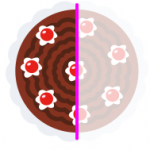Welcome back to the Smartick blog! Do you know what equivalent fractions are? After reading this post with examples of equivalent fractions, you’ll be an expert. Equivalent fractions are fractions that represent the same amount, even though they may appear to be different. Let’s look at this example: Do you think these fractions represent the […]

Aug26

## Geometric Shapes: Circle

We’ve already seen some of the main geometric shapes in earlier posts. In this post, we’re going to look more in-depth at the circle. A circle is a geometrical shape that is made by drawing a curve that always has the same distance from the point that we refer to as the center. In just […]

Aug25

## Learn How to Subtract with Examples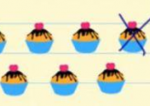In this post, we are going to learn how to subtract. First things first, we are going to learn exactly what subtraction is. Subtraction is taking away a certain amount from another amount that we already have. Some examples of how to subtract: If we have 5 purple balls in a box, and we take […]

Aug22

## How to Solve Multiplication Word Problems

Are you good at solving word problems? Solving word problems is a very important part of mathematics because with them you understand and practice what you have learned to do (adding, subtracting, multiplying…) Today we are going to look at multiplication word problems: how we recognize them, what to do to solve them…all of which […]

Aug19

## What Is a Number? Learn to Count up to 9

What Is a Number? A number helps us represent and compare quantities without having the objects in front of us. Caesar and Gabriela will share a bag of candy: Caesar says he will eat the orange candies, so Gabriela will eat the green ones. Who will eat more candy? Let’s compare Caesar’s candy with Gabriela’s: […]

Aug18

## Learn the Different Properties of Multiplication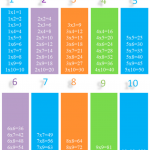In this post, we will do a review of the properties of multiplication and look at some of its applications. We begin with a brief summary of the properties of multiplication: Properties of Multiplication Commutative Property: The order of the factors does not change the product. 3 x 5 = 5 x 3 Associative Property: […]

Aug17

## I Look Like an Engineer

If there is anything certain in the debate about why there aren’t enough women in engineering and general sciences, it is that girls see too few role models in these fields. That is not to say that the availability of STEM role models is an issue that only affects girls (soccer stars and celebrities spend […]

Aug12

## How to Solve an Addition Problem with Regrouping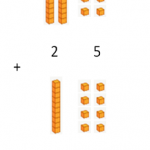Do you know how to solve an addition problem with regrouping? Do you know all the steps and why they’re important? Sometimes you learn how to solve math problems, but you don’t understand why you solve them in a particular way. This is why we are going to review how to solve an addition problem […]

Aug11

## Practice Length Measurement Problems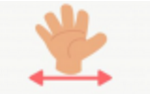To solve length measurement problems, we need to have various factors in mind: the unit of measurement used, conversion exercises, and the understanding of how to use different measuring units. In this post, we’ll present different exercise types that’ll help us understand these concepts. Unit of Measure Used to Express the Information In problems that […]